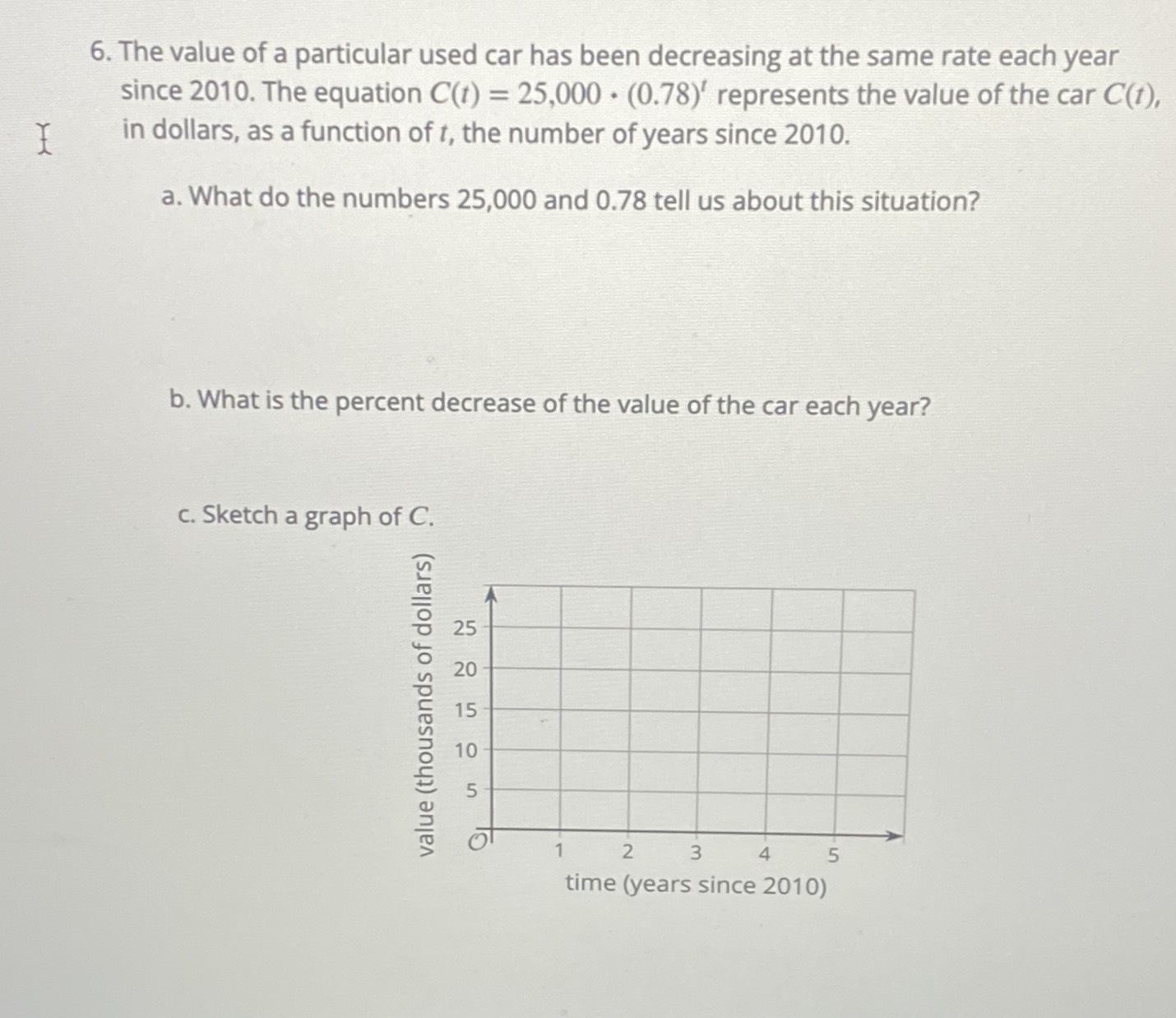### ¿Todavía tienes preguntas de matemáticas?

Pregunte a nuestros tutores expertos
Algebra
Pregunta6. The value of a particular used car has been decreasing at the same rate each year since $$2010$$ . The equation $$C ( t ) = 25,000 \cdot ( 0.78 ) ^ { t }$$ represents the value of the car $$C ( t )$$ , in dollars, as a function of $$t$$ , the number of years since $$2010$$ .

a. What do the numbers $$25,000$$ and $$0.78$$ tell us about this situation?

b. What is the percent decrease of the value of the car each year?

c. Sketch a graph of $$C$$ .

$$b.22\%$$